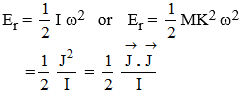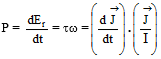# Circular Motion & Rotational Dynamics

#### 14. Kinetic Energy of Rotation

14. Kinetic Energy of Rotation
(a) The energy due to rotational motion of a body is known as rotational kinetic energy.
(b) If I be moment and inertia of body about axis of rotation and ω be its angular velocity, then kinetic energy of rotation.(c) If ω is constant, Er ∝ I
(d) If I is constant, Er ∝ ω2
(e) If J is constant, Er ∝ 1/I
(f)Work energy theorem :- The work done by torque = change in kinetic energy of rotation
(g) Power of rotation(h) If a body performs rotational kinetic motion as well as linear motion, then the total energy of body is equal to the sum of kinetic energy of rotational motion and kinetic energy of linear motion.
∴ Total K.E = Er + Et =2 +M v2
(i) If rotation is taking place without skidding then v = r ω, a = r α
(j) Unit : same as that of energy
(k) Dimension : M1 L2 T–2

If you want to give information about online courses to other students, then share it with more and more on Facebook, Twitter, Google Plus. The more the shares will be, the more students will benefit. The share buttons are given below for your convenience.

#### IIT (Class X)

• Unit, Dimension & Error
• Vectors
• Motion in One Dimension
• PROJECTILE MOTION
• NEWTON'S LAWS OF MOTION & FRICTION
• WORK, POWER, ENERGY & CONSERVATION LAWS
• CIRCULAR MOTION & ROTATIONAL DYNAMICS
• GRAVITATION